# What is the integral of dy/f'(x)?

If x = the integral of dy/f'(x) what is a x equal to? I used integration by parts. I'm trying to integrate it enough times to find a pattern (using f'''''' notation so I don't get lost). I'm having trouble doing it. If any one can spot something please share!

## Answers and Replies

Pengwuino
Gold Member
Are you asking : $$x = \int {{dy} \over {f'(x)}}$$? Well if you're integrating over dy, the function f'(x) won't be affected.

Are you asking : $$x = \int {{dy} \over {f'(x)}}$$? Well if you're integrating over dy, the function f'(x) won't be affected.
Yes, I'm trying to integrate that function. Using this method: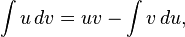u = f'(x) and dv = dy
I want to integrate this function enough times to see a pattern.

While integrating, I keep in mind that f(x) is the function of a polynomial. When integrating it is preferable to derive any f''''''(x) so that it will be possible for that derivative to reach zero.
^Sorry if this sounds confusing. It is to me.

I have tried to do integration by part twice. For example: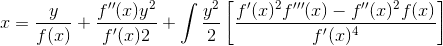I'm not very good at integrating and I can go further, but I want to know if this is correct so far.

#### Attachments

Last edited by a moderator:
HallsofIvy
Science Advisor
Homework Helper
I very much doubt you are saying what you really mean.

$$\int \frac{dy}{f'(x)}= \frac{y}{f'(x)}+ C$$
of course.

If you want to solve
$$x= \int\frac{dy}{f'(x)}$$
for x, that will depend upon exactly what f(x) is.

But I suspect you are really asking:

"By the 'Fundamental Theorem of Calculus', we know that
$$\int f'(x) dx= f(x)+ C$$

Is there a similar formula for
$$\int \frac{dx}{f'(x)}$$?"

And the answer is, "No, there isn't."

I very much doubt you are saying what you really mean.

$$\int \frac{dy}{f'(x)}= \frac{y}{f'(x)}+ C$$
of course.

If you want to solve
$$x= \int\frac{dy}{f'(x)}$$
for x, that will depend upon exactly what f(x) is.

But I suspect you are really asking:

"By the 'Fundamental Theorem of Calculus', we know that
$$\int f'(x) dx= f(x)+ C$$

Is there a similar formula for
$$\int \frac{dx}{f'(x)}$$?"

And the answer is, "No, there isn't."
Well... I found a cool trick to find the derivative of the inverse of functions

What I originally took the derivative of y:

dy/dx = f'(x)dx

Then I flipped the derivative:

dx/dy = 1/f'(x)dx If we solved for y and took the derivative of it with respect to y, you would get the answer to this equation

I then multiplied it by dy on both sides:

dx = dy/f'(x)dx

And now I hope I that I can find something out by doing the integral of this function. I'm not worrying about c because I can obtain it from from the f(x) function, which I know what it is.When I plug in f(x)= y = x^3 + x into your equation:

I get x= y/(3x^2 + 1) + c

and if I solve for y I get
y= 3x^3 + x -c
no constant can make 3x^3 + x - c = y = x^3 + x true for all real number values for x.

If sub in y = x^3 + x and f'(x) = 3x^2 + 1 I will get 0 = -2x^3 +3x^2 + 1

Hopefully I can use integration by parts to solve for x, especially with high power polynomial equations. We may be able to solve for it if we keep on doing integration by parts or any other tricks (which I don't know about) to get every thing in terms of derivatives of the function. Since you can integrate forever, I'm hopping that we can eventually reach a point were the remaining integral terms will eventually reach a integral that requires so many derivatives (for example f'''''''''''''''''''''(x)) that any polynomial can be reduced to zero, therefore "killing" the integral since it f''''''''''...''''''''(x) = 0.

I haven't considered what the bounds of the integration would be, but I'm trying to solve the integration pattern problem first. Maybe it involves some higher calculus that I haven't taken yet.

One last note, since f(x) is some polynomial, I made dx = 1 and I choose f'''''(x) notation because using an actual function would be hectic, and I wanted to see a pattern, so that I may apply this to something like x^1000 + 10x^546 + .999x^3154 .... and eventually have everything in terms of the function and it's derivatives and also so that I may take 3155 derivatives on the last integral(s) (if they exist) and kill or reduce the function to zero.

#### Attachments

Last edited by a moderator:
dy/dx = f'(x)dx

That is false.

@ homology dy/dx = f'(x)dx Your right, it is false:

dy/dx = f'(x)dx/dx = f'(x)

Still, that doesn't change the question since I originally just ignored dx (because the change in f(x) per dx or that the f'(x) is based on dx)

Char. Limit
Gold Member
It might help you more if this were written d(f(x)). Then you get...

$$\int \frac{d(f(x))}{f'(x)} = x$$

Which is an equation that makes sense.

It might help you more if this were written d(f(x)). Then you get...

$$\int \frac{d(f(x))}{f'(x)} = x$$

Which is an equation that makes sense.
Can you integrate this function (perhaps by using integration by parts)?

I know it depends on what f(x) is, but we can use general notation such as f''(x) or the integral of f(x) so that we can simply plug in for any f(x).

The point of this equation is to solve for x when f(x) is a big polynomial equation for example: f(x) = $$x^{7}$$+$$4x^{8}$$+$$2x^{23}$$+$$x^{4}$$
If we can integrate the function by parts, we may be able to put in terms of f(x) and it's derivatives.

$$\frac{dy}{dx}$$=f'(x) = $$7x^{6}$$+$$32x^{7}$$+$$64x^{22}$$+$$4x^{3}$$

$$\frac{dx}{dy}$$=$$\frac{1}{f'(x)}$$ = $$\frac{1}{[tex]32x^{7}$$+$$64x^{22}$$+$$4x^{3}$$}[/tex]

Now integrate both sides

Last edited:
Char. Limit
Gold Member
Actually, it's possible to solve this for any general function g(x). That is...

$$\int \frac{1}{f'(x)} df = g(x)$$

Can be solved for f(x). You just need to convert this integral equation into a differential equation, I believe.

disregardthat
Science Advisor
Assume f' is continuous. 1/f' wouldn't make sense if f' is zero, hence you know that since f' is continuous it is either always positive or always negative, so f is either increasing or decreasing. Thus it is bijective on its image. If the inverse is differentiable, f^(-1)'(f(x)) = 1/f'(x). Hence you want to integrate f^(-1)(f(x)) wrt f(x), but this is just f^(-1)(f(x))+C=x+C.

I have assumed two things: f' is continuous and f^(-1) is differentiable, but these things are not always true.

Last edited:
Actually, it's possible to solve this for any general function g(x). That is...

$$\int \frac{1}{f'(x)} df = g(x)$$

Can be solved for f(x). You just need to convert this integral equation into a differential equation, I believe.

$$\int \frac{1}{f'(x)} df = \int \frac{f'(x)}{f'(x)} dx = x + C$$

So, g(x) = x for C^1 f.

Char. Limit
Gold Member
$$\int \frac{1}{f'(x)} df = \int \frac{f'(x)}{f'(x)} dx = x + C$$

So, g(x) = x for C^1 f.

Yeh, my bad. I trusted the online too much.

Assume f' is continuous. 1/f' wouldn't make sense if f' is zero, hence you know that since f' is continuous it is either always positive or always negative, so f is either increasing or decreasing. Thus it is bijective on its image. If the inverse is differentiable, f^(-1)'(f(x)) = 1/f'(x). Hence you want to integrate f^(-1)(f(x)) wrt f(x), but this is just f^(-1)(f(x))+C=x+C.

I have assumed two things: f' is continuous and f^(-1) is differentiable, but these things are not always true.
I am dealing with polynomials (and other functions) which are continuous and always differentiable.

Actually, it's possible to solve this for any general function g(x). That is...

$$\int \frac{1}{f'(x)} df = g(x)$$

Can be solved for f(x). You just need to convert this integral equation into a differential equation, I believe.
If g(y) = x and f(x) = y, then I thought that g'(y) = dx/dy, since f(x) = dy/dx
so the integral of dx/dy + C= g(x)

What I think you are saying is g(y) = the integral of (dx/dy)(dy/dx) + C then shouldn't the (dx/dy)(dy/dx) = 1?

$$\int \frac{1}{f'(x)} df = \int \frac{f'(x)}{f'(x)} dx = x + C$$

So, g(x) = x for C^1 f.
Just for clarification, we know the function -f(x)- is, so we can find the integral constant. Are we able to integrate the derivative of the inverse of f(x) or what you call g'(y) [or g'(x) I don't know which] to find what the function g(x) is?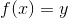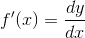g(y) is the inverse of f(x)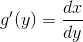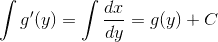Now, the practical purpose question: If f(x) is some huge polynomial, then can we find what g(y) is equal to?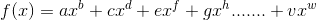Every letter is a constant except x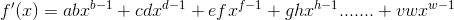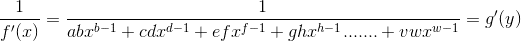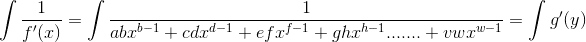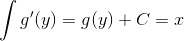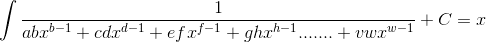Now here is what I want to really know. Can we solve this integral? and if we can how? and even then, what are it's bounds?

Edit: I would also like to know the same thing if I simply added a constant after vx^w + z to the equations. Think that if you do integration by parts, all the remaining integrals can eventually be in terms of f''''''''''''''.....''''''''(x) = 0 so that the integral eventually"disappears" <-please quote the edit if I'm not being clear.

Last edited by a moderator: